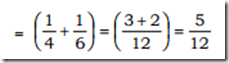## Tuesday, 27 September 2016

In  most  of  the  problems  based  on  Time  and  Work, either  the  amount  of  time  taken  to  finish  a  given job  or  the  amount  of  work  done  is  to  be  calculated. Unless  otherwise  specified,  the  amount  of  work done  is  generally  taken  as  unity  (1).  Also  if  it  is given  that  a  person  (P)  can  finish  a  job  in  D  days, then  it  implies  that  P  alone  can  do  the  job  in  D days.

Important Points to Remember :

(i) Capacity  of  persons  to  do  a  piece  of  work  is always  constant.  That  is,  the  person  does the  equal  work  every  day.

(ii) In  the  question  based  on  ‘Time  and  Work’, generally  we  find  amount  of  work  done  in unit  time  (1  day,  1  hour  etc).  Thus,  if  a man  can  do  a  piece  of  work  in  x days  (or hours  or  any  other  unit  of  time),  then  the work  done  by  him  in  one  day  will  be(1\x) of  the  total  work

(iii) If  A  is  twice  as  good  as  a  workman  as  B, then  A  will  take  half  the  time  B  takes  to finish  a  piece  of  work.

(iv) Application  of  the  Concept  of  Unitary Method  and  Variation: Since  in  problems on  Time  and  Work,  there  is  a  proportional relation,  hence  we  solve  them  by  Unitary Method  and  Ratio  and  Proportion

(v) If  to  finish  a  certain  piece  of  work,  some men  are  employed  and  they  finish  the  work in  a  certain  time,  then  the  relation  between work,  man  and  time  will  be  as  follows:

(a) Work  and  Man: Number  of  persons employed  to  do  the  work  is  directly proportional to  the  amount  of  work done.  (More  t he  number  of  personsemployed,  more  the  work  done)

(b) Time  and  Work: The  number  of  days is  directly  proportional to  work  done. (More  the  number  of  days  for  which  a work  was  done,  more  shall  be  the  total amount  of  work  done)

(c) Time  and  Man: The  number  of  persons employed  is  inversely  proportional to the  number  of  days  required  to  finish  a work.  (More  the  number  of  persons employed,  less  will  be  the  time  required to  finish  the  work)

Example :

1: Amit  can  do  a  piece  of  work  in  4  days and  Sumit  can  do  it  in  6  days.  How long  will  they  take,  if  both  Amit  and Sumit  work  together?

We  have,  time  taken  by  Amit  to  do  the work  =  4  days
Time  taken  by  Sumit  to  do  the  work =  6  day

 Work  done  by  Amit  in  1  day  = 1/4

Work  done  by  Sumit  in  1  day = 1\6

So,  work  done by  Amit  and  Sumit in  1  dayHence,  Amit  and  Sumit  can  do  the  piece of  work  in 12\5 days i.e 2(5/12)